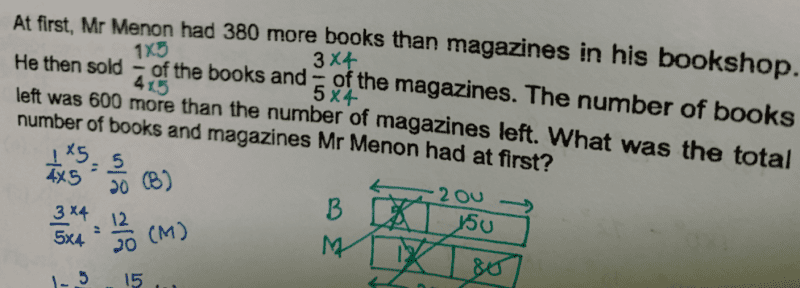# QuestionBooks     [———–u—————]

Mag        [———–u—————]

Books left = 3/4 = 15/20

Mags left = 2/5 =  8/20

(15/20)(u+380) – (8/20)u = 600

15u + 5700 – 8u = 600 x 20

7u = 12000-5700 = 6300

u = 900

Therefore, total books and mags at first = 2u + 380 = 2(900)+380 = 2180

Thanks!  Here’s how I did it through equations:

B – M = 380
B = 380 + M … (1)

(3/4)B – (2/5)M = 600
B – (4/3)(2/5)M = (4/3)600 = 800
B = 800 + (8/15)M … (2)

Placing (1) into (2):
380 + M = 800 + (8/15)M
(7/15)M = 800 – 380 = 420
M = (15/7)420 = 900

Therefore B = 380 + 900 = 1280

So total books and magazines he had at first = 900 + 1280 = 2180 #

0 Replies 0 Likes